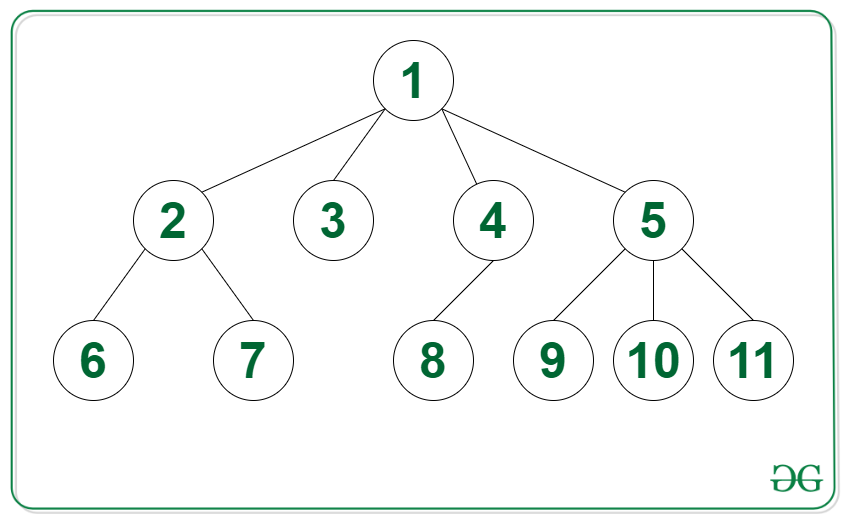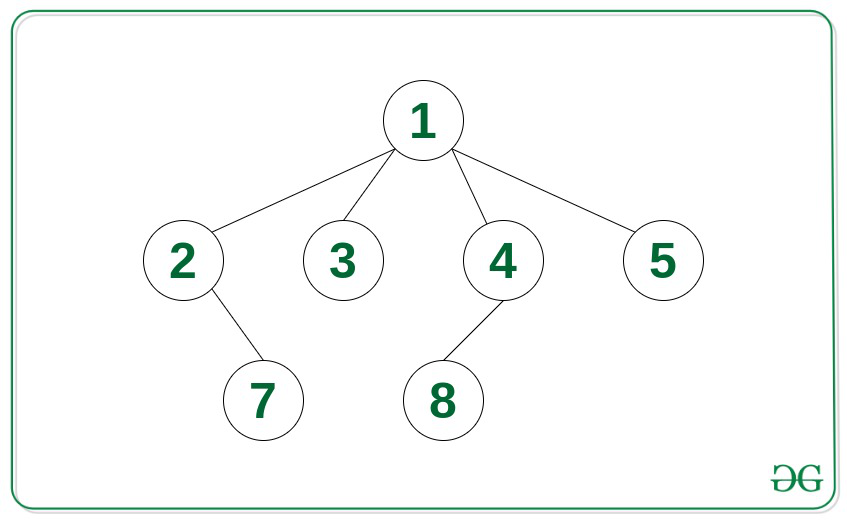# Level order traversal by converting N-ary Tree into adjacency list representation with K as root node

• Last Updated : 01 Oct, 2021

Given the root node of an N-ary tree and an integer K, the task is to convert the given tree into adjacency list representation and print the level order traversal considering vertex K as the root node.

Example:

Input: Tree in the image below, K = 5Output:

1 9 10 11
2 3 4
6 7 8

Input: Tree in the image below, K = 5Output:

1
2 3 4
7 8

## Recommended: Please try your approach on {IDE} first, before moving on to the solution.

Approach: The given problem can be solved by using the DFS Traversal on the N-ary tree and storing the relation of all the edges into an adjacency list according to the adjacency list representation. The created adjacency list can be used to print the Level Order Traversal with K as the root node. This can be done using BFS traversal which is discussed in this article.

Below is the implementation of the above approach:

## C++

 `// C++ program for the above approach ` `#include ` `using` `namespace` `std; ` ` `  `// A binary tree node ` `struct` `Node { ` `    ``int` `data; ` `    ``vector child; ` `}; ` ` `  `// Function to create a new tree node ` `Node* newNode(``int` `key) ` `{ ` `    ``Node* temp = ``new` `Node; ` `    ``temp->data = key; ` `    ``return` `temp; ` `} ` ` `  `// Adjacency list to store the Tree ` `vector > adj; ` ` `  `// Function to perform the DFS traversal ` `// of the N-ary tree using the given ` `// pointer to the root node of the tree ` `void` `DFS(``struct` `Node* node) ` `{ ` `    ``// Traverse all child of node ` `    ``for` `(``auto` `x : node->child) { ` `        ``if` `(x != NULL) { ` ` `  `            ``// Insert the pair of vertices ` `            ``// into the adjacency list ` `            ``adj[node->data].push_back(x->data); ` `            ``adj[x->data].push_back(node->data); ` ` `  `            ``// Recursive call for DFS on x ` `            ``DFS(x); ` `        ``} ` `    ``} ` `} ` ` `  `// Function to print the level order ` `// traversal of the given tree with ` `// s as root node ` `void` `levelOrderTrav(``int` `s, ``int` `N) ` `{ ` `    ``// Create a queue for Level ` `    ``// Order Traversal ` `    ``queue<``int``> q; ` ` `  `    ``// Stores if the current ` `    ``// node is visited ` `    ``vector<``bool``> visited(N); ` ` `  `    ``q.push(s); ` ` `  `    ``// -1 marks the end of level ` `    ``q.push(-1); ` `    ``visited[s] = ``true``; ` `    ``while` `(!q.empty()) { ` ` `  `        ``// Dequeue a vertex from queue ` `        ``int` `v = q.front(); ` `        ``q.pop(); ` ` `  `        ``// If v marks the end of level ` `        ``if` `(v == -1) { ` `            ``if` `(!q.empty()) ` `                ``q.push(-1); ` ` `  `            ``// Print a newline character ` `            ``cout << endl; ` `            ``continue``; ` `        ``} ` ` `  `        ``// Print current vertex ` `        ``cout << v << ``" "``; ` ` `  `        ``// Add the child vertices of ` `        ``// the current node in queue ` `        ``for` `(``int` `u : adj[v]) { ` `            ``if` `(!visited[u]) { ` `                ``visited[u] = ``true``; ` `                ``q.push(u); ` `            ``} ` `        ``} ` `    ``} ` `} ` ` `  `// Driver Code ` `int` `main() ` `{ ` `    ``Node* root = newNode(1); ` `    ``(root->child).push_back(newNode(2)); ` `    ``(root->child).push_back(newNode(3)); ` `    ``(root->child).push_back(newNode(4)); ` `    ``(root->child).push_back(newNode(5)); ` `    ``(root->child->child).push_back(newNode(6)); ` `    ``(root->child->child).push_back(newNode(7)); ` `    ``(root->child->child).push_back(newNode(8)); ` `    ``(root->child->child).push_back(newNode(9)); ` `    ``(root->child->child).push_back(newNode(10)); ` `    ``(root->child->child).push_back(newNode(11)); ` `    ``int` `N = 11; ` `    ``int` `K = 5; ` `    ``adj.resize(N + 1, vector<``int``>()); ` ` `  `    ``DFS(root); ` `    ``levelOrderTrav(5, 11); ` ` `  `    ``return` `0; ` `}`

Output:

```5
1 9 10 11
2 3 4
6 7 8
```

Time Complexity: O(N)
Auxiliary Space: O(N)

My Personal Notes arrow_drop_up
Recommended Articles
Page :